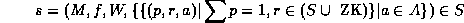Next: Notation and Formalisms Up: Using Recursive Agent Models Previous: The Pursuit Task# Theory

The basic unit in our syntactical framework for recursive agent modeling is the situation. At any point in time, an agent is in a situation, and all the other agents present are in their respective situations. An agent's situation contains not only the situation the agent thinks it is in but also the situations the agent thinks the others are in (these situations might then refer to others and so on ...). In this work, we have adopted RMM's assumption that common knowledgecannot arise in practice. Common knowledge would be represented in our framework by having situations refer back to themselves either directly or transitively. Conversely, cycles or loops of reference between situations imply the presence of common knowledge. They are, therefore, disallowed since common knowledge can not be achieved in many common situations of interest to us, such as in asynchronous message-passing system . Allowing agents to jump to assumptions about common knowledge can simplify coordination reasoning, since agents can use fixed-point equilibrium notions and axioms based on mutual beliefs and plans. While leaping to such assumptions can, at times, be a viable method for keeping coordination practical , it also introduces risks that we wish to avoid.

A situation has both a physical and a mental component. The physical component refers to the physical state of the world and the mental component to the mental state of the agent, i.e. what the agent is thinking about itself and about the other agents around it. Intuitively, a situation reflects the state of the world from some agent's point of view by including what the agent perceives to be the physical state of the world and what the agent is thinking. A situation evaluates to a strategy, which is a prescription for what action(s) the agent should take. A strategy has a probability associated with each action the agent can take, and the sum of these probabilities must always equal 1.

Let S be the set of situations an agent might encounter, and A the set of all other relevant agents. A particular situation s is recursively defined as:The matrix M has the payoff the agent, in situation s, expects to get for each combination of actions that all the agents might take. The matrix M can either be stored in memory as is, or it can be generated from a function (which is stored in the agent's memory) that takes as inputs any relevant aspects of the physical world, and previous history. The relevant aspects of the physical world are stored in W, the physical component of the situation. The rest of s constitutes the mental component of the situation.

The probabilistic distribution function f(x) gives the probability that the strategy x is the one that the situation s will evaluate to. It need not be a perfect probabilistic distribution; it is merely used as a useful approximation for guiding our search through the recursive models. That is, our algorithm will employ it as a heuristic for determining which recursive models to expand. We use f(x) to calculate the strategy that the agent in the situation is expected to choose, using standard expected value formulas from probability theory. The values of this function are usually calculated from previous experience. In practice, some simplifying assumptions can be made about how to calculate (i.e. learn) this value, as we shall explain in Section 3.2. We also note that the knowledge encompassed by f(x) is not the real knowledge, which is contained in the matrices, but is the search knowledge used only for guiding the search through the recursive models. There is no guarantee that the predictions produced by f(x) will be correct. The models, on the other hand, are always considered to be correct.

A situation s also includes the set of situations which the agent in s believes the other agents are in. Each agent a is believed to be in r, with probability p. The value of r can either be a situation or, if the modeling agent has no more knowledge, it can be the Zero Knowledge strategy (r = ZK).Next: Notation and Formalisms Up: Using Recursive Agent Models Previous: The Pursuit Task

Jose M. Vidal
jmvidal@umich.edu
Sun Mar 10 12:52:06 EST 1996